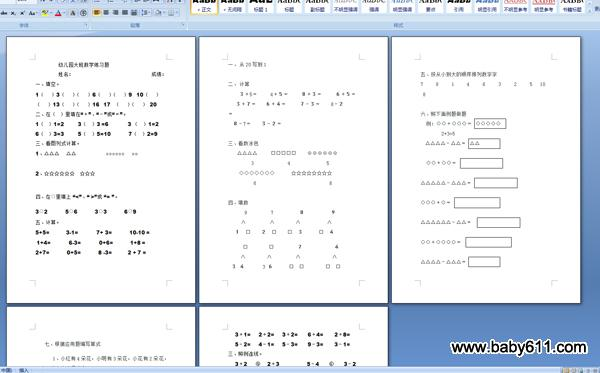# 幼儿园学前班大班数学测试题姓名：                               成绩：

一、填空。

1（    ）3（    ）（    ）6（    ）（    ）9  10（    ）

（    ）13（    ）（    ）16  17  （    ）（    ） 20

二、在（  ）里填在"＋"、"－"或"＝"。

1（  ）1=2        3（  ）3 =6          3 （  ）1=2

6（  ）3=3       5（  ）5=10         7（  ）2=9

三、看图列式计算。

1、△△△    △△                 ○○○○○○    ○○

2、☆☆☆☆☆☆   ☆☆☆

四、在○里填上 "<"、" >"或 "= "。

3○2          5○6          3○3          6○9

五、计算。

5+5=          3-1=          7+ 3=          10-10 =

1+4=          6-3=          0+6=          1+8 =

2+7=          0+5=          8 -3=         2 + 7 =

一、从20写到1

二、计算

３＋5＝    4＋５＝    ８＋３＝　　 ６＋５＝

３＋７＝　   ６＋４＝　   ７－３＝　  8－２＝

８－7＝　　３－２＝

三、看数涂色

△△△△ 　　□□□□□　　⊙⊙⊙⊙⊙⊙

3 　　　　　　4 　　　　　  5

◇◇◇◇◇◇◇　　　☆☆☆☆☆☆☆☆

6 　　　　　         8

四、填数

9　　　　 7　　　　  ８　　　　　９

∧　　　　 ∧　　　　∧　　　　　∧

１　□　　２　□　　□　３　　　□　４

□　　　  □　　　　 ７　　　　　　４

∧　　　  ∧　　　　 ∧　　　　　　∧

３ ４　　  3 ６　　  □　□　　　　□　□

五、按从小到大的顺序排列数字字　7   9   1   4   6   3   2   5  10  8

六、照下面例题做题

例：○○＋○○○＝ ○○○○○

2+3=5

△△△△－△△＝ △△

○○＋○○＝

△△△△△－△△△＝

○○○＋○＝

△△△△△△－△△＝

○○＋○○○○＝

△△△△－△△△＝

七、根据应用题编写算式

1、小红有4朵花，小明有3朵花，小花有2朵花，三人一共有多少朵花？

2、小明买了5支铅笔，用了3支，小明还有多少支？

3、小华家有4口人，小刚家比小华家多2口人，小刚家有几口人？

一、在方格里填上合适的数。

6         2        3         4         5

／＼      ／＼     ／＼      ／＼      ／＼

□   3     □   1     □  2      3  □      4  □

□         □        □        □         □

／＼      ／＼      ／＼      ／＼       ／＼

2   1     2   2      2  5      5  3      5   5

二、计算。

3＋1=     2＋2=     3＋2=      6＋4=       2＋8=

5－2=     4－1=     5－3=      9－3=       3－1=

三、照例连线。

3＋2     ④    2＋3              5－4      ⑥      3－2

1＋1     ⑤    6－4              2＋4      ②      4＋2

4－3     ②    4－3              1＋1      ⑤      4－2

3＋3     ①    2＋4              2＋3      ④      2＋3

2＋2     ⑥    3＋1              6－2      ①      2＋2

四、给正确答案后"√"，错误的"×"。

2＋4=5(×)     2＋1=3(   )      1＋4=5(   )

3＋1=4(   )    3－2=2(   )      2＋3=5(   )

2＋3=4(   )    4－1=3(   )      2－1=2(   )

1＋4=5(   )    3＋2=4(   )      3－2=2(   )

五、看图写算式：

○○○●●              □□■            △△▲▲▲▲

(   )＋(   )=         (   )＋(   )=        (   )＋(   )=

(   )＋(   )=         (   )＋(   )=        (   )+(   )=

(   )－(   )=         (   )－(   )=        (   )-(   )=

• 幼儿园试卷分类
• 将此试卷分享到：
• 大班试卷最新更新